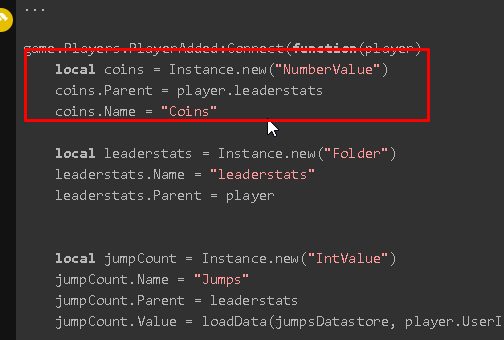Hello, I need help adding a second leaderstats named “Coins” appear on the leaderboard along with the first one which is “Jumps”. I’m by no means an expert scripter, I tried to do something but it just renames the “Jumps” leaderstats and doesn’t make the script work.

Below I’m leaving the original script:

``````local DataStoreService = game:GetService("DataStoreService")
local jumpsDatastore = DataStoreService:GetDataStore("JumpsDataStore")

local success, data = pcall(function()
return datastore:GetAsync(key)
end)

return data
end

local function saveData(datastore, key, value)
local success, err = pcall(function()
datastore:SetAsync(key, value)
end)

if err then print(err) end
end

local jumpCount = Instance.new("IntValue")
jumpCount.Name = "Jumps"
jumpCount.Value = loadData(jumpsDatastore, player.UserId) or 0

local humanoid = character:WaitForChild("Humanoid")
local debounce = true

humanoid:GetPropertyChangedSignal("Jump"):Connect(function()
if debounce == true then
debounce = false
if humanoid.Jump == true then
jumpCount.Value = jumpCount.Value + 1
end
wait(0.2)
debounce = true
end
end)
end)
end)

game.Players.PlayerRemoving:Connect(function(player)
saveData(jumpsDatastore, player.UserId, jumps.Value)
end)
``````

Thanks in advance to anyone trying to helpJust add another number value using `Instance.new()`

``````-- Code
local coins = Instance.new("NumberValue")
coins.Name = "Coins"
``````

Simple as that.

``````...

local coins = Instance.new("NumberValue")
coins.Name = "Coins"

local jumpCount = Instance.new("IntValue")
jumpCount.Name = "Jumps"
jumpCount.Value = loadData(jumpsDatastore, player.UserId) or 0

...
``````

but now it does not display any leaderstats. Sorry for the stupid question and issueYou are putting it before leaderstats is creating.``````...

local coins = Instance.new("NumberValue")